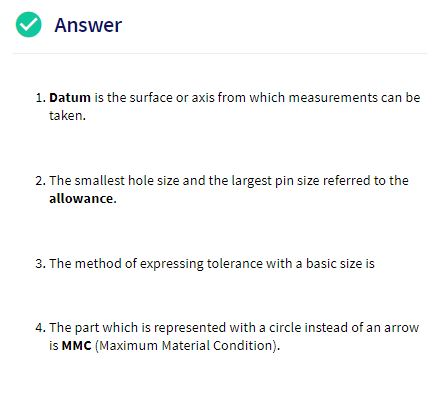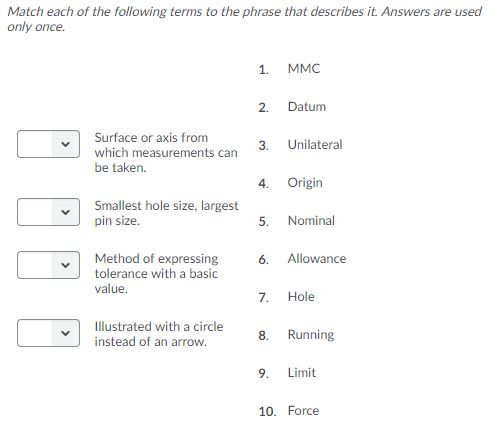# Answer 1. Datum is the surface or axis from which measurements can be taken The smallest hole size and the largest pin size referred to the allowance. 3. The method of expressing tolerance with a basic size is 4. The part which is represented with a circle instead of an arrow is MMC (Maximum Material Condition) Match each of the following terms to the phrase that describes it. Answers are used only once. МMС 1 Datum 2. Surface or axis from Unilateral 3. which measurements can be taken. Origin 4. Smallest hole size, largest pin size. Nominal 5. Method of expressing tolerance with a basic value. Allowance 6. Hole 7. llustrated with a circle instead of an arrow. Running 8 9 Limit 10. Force

Question

Match the following term to the phrase that describes it.

I had this question answered but number 3 was left unanswered. Please solve number 3 for me: "Method of expressing tolerance with a basic value".help_outlineImage TranscriptioncloseAnswer 1. Datum is the surface or axis from which measurements can be taken The smallest hole size and the largest pin size referred to the allowance. 3. The method of expressing tolerance with a basic size is 4. The part which is represented with a circle instead of an arrow is MMC (Maximum Material Condition) fullscreenhelp_outlineImage TranscriptioncloseMatch each of the following terms to the phrase that describes it. Answers are used only once. МMС 1 Datum 2. Surface or axis from Unilateral 3. which measurements can be taken. Origin 4. Smallest hole size, largest pin size. Nominal 5. Method of expressing tolerance with a basic value. Allowance 6. Hole 7. llustrated with a circle instead of an arrow. Running 8 9 Limit 10. Force fullscreen